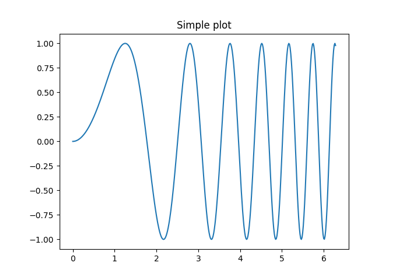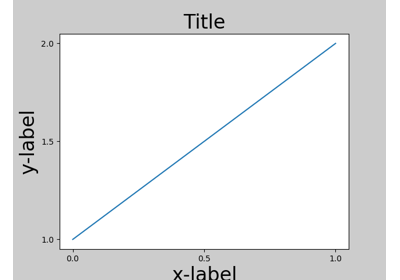Travis-CI:# matplotlib.pyplot.close¶

`matplotlib.pyplot.``close`(*args)

Close a figure window.

`close()` by itself closes the current figure

`close(h)` where h is a `Figure` instance, closes that figure

`close(num)` closes figure number num

`close(name)` where name is a string, closes figure with that label

`close('all')` closes all the figure windows

## Examples using `matplotlib.pyplot.close`¶Subplots DemoPipongMultipage PDFMultiprocessTight Layout guide